# Torsion

Introduction..... Nomenclature..... Formulas..... Sections _ Structural Engineering.....

Introduction

A bar of uniform section fixed at one end and subject to a torque at the extreme end which is applied normal to its axis will twist to some angle which is proportional to the applied torque.     This assumes that the bar is not stressed to a level greater than its elastic limit.     This page includes various formulas which allow calculation of the angles of twist and the resulting maximums stresses. The equations are based on the following assumptions

1) The bar is straight and of uniform section
2) The material of the bar is has uniform properties.
3) The only loading is the applied torque which is applied normal to the axis of the bar.
4) The bar is stressed within its elastic limit.

Nomenclature

T = torque (Nm)
l = length of bar (m)
J = Polar moment of inertia.(Circular Sections) ( m4 )
J' = Polar moment of inertia.(Non circluar sections) ( m4 )
K = Factor replacing J for non-circular sections.( m4 )
r = radial distance of point from center of section (m)
ro = radius of section OD (m)
τ = shear stress (N/m2)
G Modulus of rigidity (N/m2)

Formulas

Formulas for bars of circular section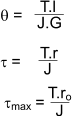Formulas for bars of non - circular section.

Bars of non -circular section tend to behave non-symmetrically when under torque and plane sections to not remain plane. Also the distribution of stress in a section is not necessarily linear.

The general formula of torsional stiffness of bars of non-circular section are as shown below the factor J' is dependent of the dimensions of the section and some typical values are shown below.    For the circular section J' = J.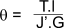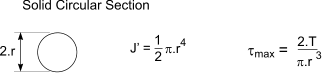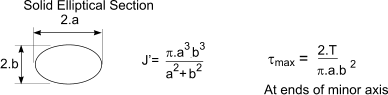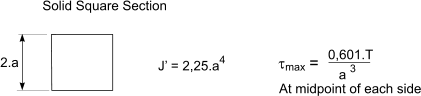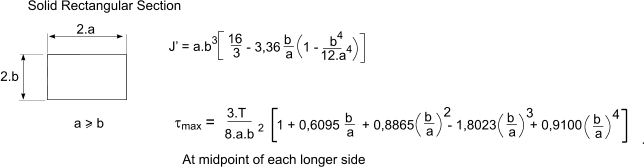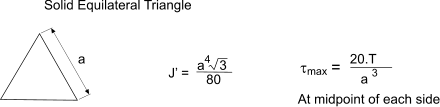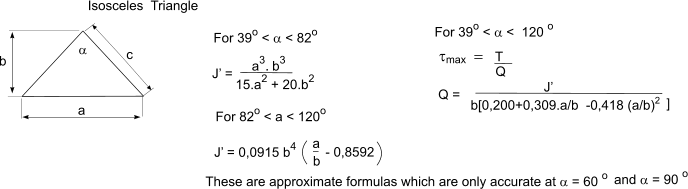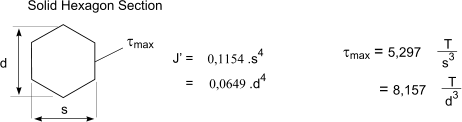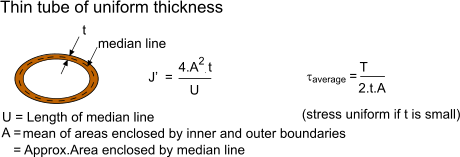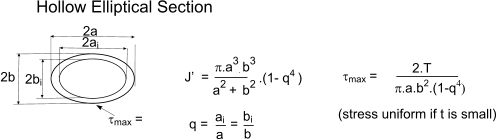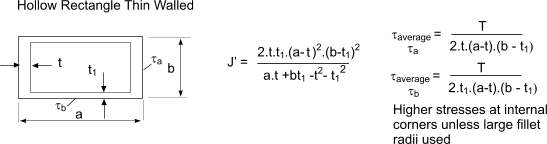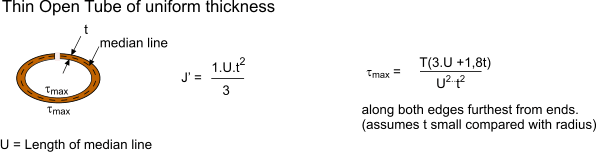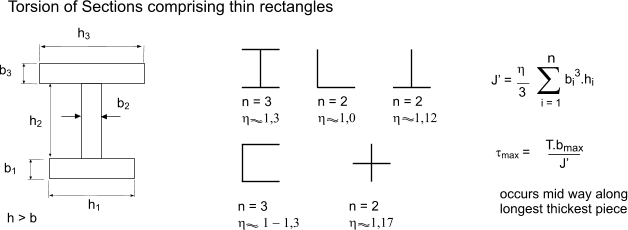Testing the values of J' obtained using the above equations (with η = 1) with the values obtained from the table below the following values result.

1)Channel section 430x100x64 calculated J' = 63,118 cm4. Table value 63     η = 1
2) Channel section 100x50x10 calculated J' = 2,39 cm4. Table value 2,53     η = 1,06
3)Tee section 305x457x127 calculated J' = 296 cm4. Table value 312     η = 1,06
4)Tee section 133x102x137 calculated J' = 2.69 cm4. Table value 2,97     η = 1,10

Torsion in Sections. Structrual design

Important Note : In the notes and tables below J is used throughout for the torsion constant for circular and non circular sections. .
This is the convention in structural design

In structural design the use of sections i.e I sections, channel section, angle sections etc. should be avoided for applications designed to withstand torsional loading.     Hollow rectangular sections are best suited for these applications.    Note: Values for J and C for square and hollow rectangular sections are provided on webpages as indexed on webpage Sections Index

 In the steel Sections tables i.e BS EN 10210-2: 1997"Hot finished Rectangular Hollow Sections" & BS EN 10219-2:"Cold Formed Circular Hollow Sections" The Torsion Constant J and the Torsion modulus constant C are listed. These are calculated as follows. The Torsion constant (J) for Hollow Rolled Sections are calculated as follows: For circular hollow sections.... J = 2I For square and rectangular hollow sections ...... J = 1/3 t3h + 2k Ah where: I is the second moment of area t is the thickness of section h is the mean perimeter = 2 [(B - t) + (D - t)] - 2 Rc (4 - p) Ah is the area enclosed by mean perimeter = (B - t) (D - t) -Rc2 (4 - p) k =2 Ah t / h B is the breadth of section D is the depth of section Rc is the average of internal and external corner radii. Torsion modulus constant C For circular hollow sections.............C = 2.Z Z = elastic modulus = J /r For square and rectangular hollow sections.............C = J / ( t + k / t ) Note: Values for J and C for square and hollow rectangular sections are provided on webpages as indexed on webpage Sections Index

Torsional /Buckling Properties for Hot rolled Sections

A few tables providing Torsional /Buckling properties for some steel sections are indexed below.

 Universal Beams Universal beams Torsion/Buckling Properties BS 4:-1  2005) Universal Columns Universal Columns Torsion/Buckling Properties BS 4 Part 1: 2005 Rolled Steel Joists Joists Torsion/Buckling Properties BS 4 Part 1: 2005 Channels Channels Torsion/Buckling Properties BS 4 Part 1: 2005 Channels-Parallel Flanged Parallel Flanged Channels Torsion/Buckling Properties BS 4 Part 1: 2005 Tees cut for Universal columns Tees from UC Torsion/Buckling Properties BS 4 Part 1: 2005 Tees cut for Universal Beams Tees from UB Torsion/Buckling Properties BS 4 Part 1: 2005 Tees cut from Joists Tees from Joists /Buckling Properties BS 4 Part 1: 2005